# Test: Level - 2 Laws Of Motion - 1

## 30 Questions MCQ Test Physics Class 11 | Test: Level - 2 Laws Of Motion - 1

Description
This mock test of Test: Level - 2 Laws Of Motion - 1 for Class 11 helps you for every Class 11 entrance exam. This contains 30 Multiple Choice Questions for Class 11 Test: Level - 2 Laws Of Motion - 1 (mcq) to study with solutions a complete question bank. The solved questions answers in this Test: Level - 2 Laws Of Motion - 1 quiz give you a good mix of easy questions and tough questions. Class 11 students definitely take this Test: Level - 2 Laws Of Motion - 1 exercise for a better result in the exam. You can find other Test: Level - 2 Laws Of Motion - 1 extra questions, long questions & short questions for Class 11 on EduRev as well by searching above.
QUESTION: 1

### A man getting down a running bus, falls forward because-

Solution:

The explanation is the question itself. inertia is a property of which it resists its change of state of rest or state of motion. so as soon as the person jumps the lower part immediately comes to rest by sharing contact with the ground the upper body due to inertia of motion resists its change in state of motion

QUESTION: 2

### You are on a friction less horizontal plane. How can you get off if no horizontal force is exerted by pushing against the surface ?

Solution:

Spitting or sneezing this is because you can not jump foreward or backward or rolling or even run on the frictionless plane. So you have to throw anything out of your body by applying force third laws follows here and you will escape out.

QUESTION: 3

### The forces acting on an object are shown in the fig. If the body moves horizontally at a constant speed of 5 m/s, then the values of the forces P and S are, respectively-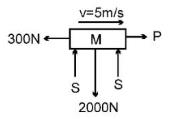Solution:

As there is no net acceleration in horizontal or vertical direction, we can say that
P = 300N
And 2S = 2000N
Thus S = 1000N

QUESTION: 4

A boy of mass 50 Kg running at 5 m/s jumps on to a 20Kg trolley travelling in the same direction at 1.5 m/s. What is the common velocity?

Solution:

Since no external force, law of
conservation of momentum can be applied Initial Momentum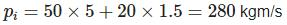Final momentum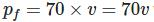where v is the common velocity
Now we know that
Initial momentum=Final momentum
280 = 70v
V=4m/s

QUESTION: 5

Find the velocity of the hanging block if the velocities of the free ends of the rope are as indicated in the figure.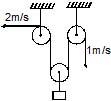Solution: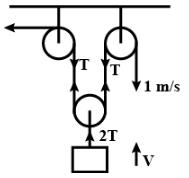Workdone by T=0
∴∑T⋅V=0
2⋅T+1⋅T+2TV=0
⇒−3T=−2TV (T & 2 are 180o)
∴V=3/2 m/s↑

QUESTION: 6

At a given instant, A is moving with velocity of 5 m/s upwards. What is velocity of B at the time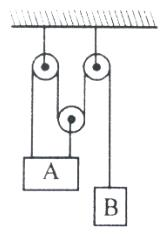Solution:

l1=2l2+l3= constant
dl1/dt+2dl2/dt+dl3/dt=0
−5+2(−5)+dl/dt=0
dl3/dt=15m/s
⇒vB=15m/s↓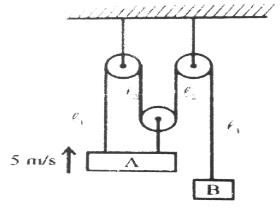QUESTION: 7

A body of mass 5 kg is suspended by the strings making angles 60º and 30º with the horizontal -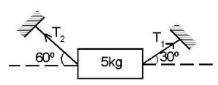(a) T1 = 25 N

(b) T2 = 25 N

(c) T1 = 25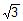N

(d) T2 = 25N

Solution:

As the mass is at rest the resultant of forces acting on it are equal to zero

so forces in vertical direction are

T1​sin30o+T2​sin60o−mg=0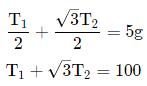similarly in horizontal direction

T1​sin30o−T2​sin60o=0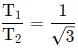solving above equations will give us

T1​+√3​×√3​T1​=100

T1​=25N

T2​=25√3​N

QUESTION: 8

A cyclist of mass 30 kg exerts a force of 250 N to move his cycle. acceleration is 4 ms−2. force of friction between road and tyres will be

Solution:
QUESTION: 9

In the arrangement shown in fig. the ends P and Q of an unstretchable string move downwards with uniform speed U. Pulleys A and B are fixed. Mass M moves upwards with a speed.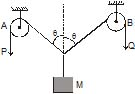Solution: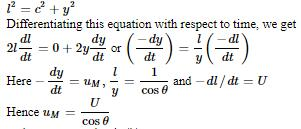Thus option D is correct

QUESTION: 10

The velocity of end `A' of rigid rod placed between two smooth vertical walls moves with velocity `u' along vertical direction. Find out the velocity of end `B' of that rod, rod always remains in constant with the vertical walls.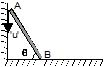Solution:

Let say end b has some velocity v in horizontal direction. Thus by constraint motion we get the component of velocities along the rod of both the ends must be equal thus we get,
u.cos (90-q) = v.cos q
Thus we get v = u.tan q

QUESTION: 11

A cyclist of mass 30 kg exerts a force of 250 N to move his cycle. The acceleration is 4 ms−2. The force of friction between the road and tyres will be

Solution:

As 250N is applied to move the cyclist and cycle, and lets say some frictional force f is acting upon it.
Thus we get 250 - f = 30 x 4 =120
Thus we get f = 130N

QUESTION: 12

Find out the reading of the weighing machine in the following cases.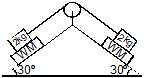Solution:

N = mgcosФ
= 2 x 10 x cos30
=2 x 10 x root 3/2     = 10 root3 N

QUESTION: 13

A mass M is suspended by a rope from a rigid support at A as shown in figure. Another rope is tied at the end B, and it is pulled horizontally with a force F. If the rope AB makes an angle q with the vertical in equilibrium, then the tension in the string AB is :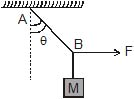Solution:

As the block is still at the equilibrium, we get
T.cos q= mg
T.sin q= F
Thus we T = F / sin q

QUESTION: 14

Three block are connected as shown, on a horizontal frictionless table and pulled to the right with a force T3 = 60 N. If m1 = 10 kg, m2 = 20 kg and m3 = 30 kg, the tension T2 is-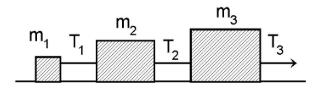Solution:

Let a be the acceleration of the system.
T1​=M1​a .....(1)
T2​−T1​=M2​a ....(2)
F−T2​=M3​a ......(3)
Adding (1), (2) and (3)  we get
(M1​+M2​+M3​)a=F
or  (10+20+30)a=60⇒a=1m/s2
Now , T2​=(M1​+M2​)a=(10+20)(1)=30N

QUESTION: 15

Three blocks A, B and C are suspended as shown in the figure. Mass of each blocks A and C is m. If system is in equilibrium and mass of B is M, then :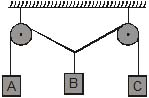Solution: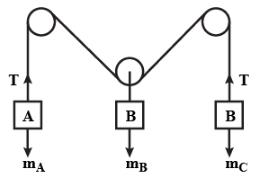Given,
Mass of block A & B =m
Mass of block C =M
Let
Mass of A =mA​ & Mass of B =mB
Tension in string is =T
At equilibrium, T=mA​g=mB​g=mg
Weight of block C is = Mg

Forces on block C
2Tcosθ=Mg
cosθ=Mg/2T=Mg/2mg ​=M/2m
If 0<θ<90° then 1>cosθ>0
1>M/2m >0
2m>M
Hence, 2m>M

QUESTION: 16

A weight can be hung in any of the following four ways by string of same type. In which case is the string most likely to break ?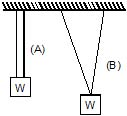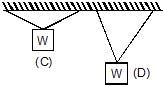Solution:

In all the given cases the cos component of the tension in the string would balance the weight of the block while the sin component will cancel themselves as they are in pair.
The larger would be the angle the smaller would be the cos component but as its value is fixed i.e. is mg,  we get the larger the angle the larger the tension and hence the more chances of breaking of the rope.

QUESTION: 17

A force-time graph for a linear motion is shown in figure where the segments are circular. The linear momentum gained between zero and 8 seconds in -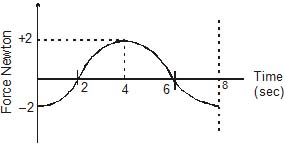Solution:

Momentum is rate of change of force according to the given graph initial and final forces are same ie, no change in force therefore b/w 0 and 8 seconds linear momentum is 0.

QUESTION: 18

A particle moves in the xy plane under the action of a force F such that the value of its linear momentum (P) at any time t is, Px = 2 cost, Py = 2 sint. The angle q between P and F at that time t will be -

Solution: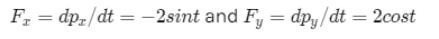So angle between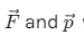will be 90º  because we see that their dot product is zero.

QUESTION: 19

A stunt man jumps his car over a crater as shown (neglect air resistance)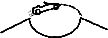Solution:

When the man is inside the car he experience the acceleration as that of car that's what man experience weightlessness. It is similar to spacecraft concept. When the space craft comes to earth people inside it experience same acceleration and feel weightless

QUESTION: 20

A particle of mass 50 gram moves on a straight line. The variation of speed with time is shown in figure. find the force acting on the particle at t = 2, 4 and 6 seconds.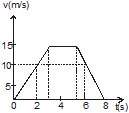Solution:

Mass of the particle = 50 g = 0.05 kg
To know the force acting on the particle, we need to know the acceleration of the particle. In the velocity-time graph the slope of the curve gives the instantaneous acceleration. Let us find it for the given instant.
At t=2 seconds
The graph is a straight line with positive slope. It means the particle has a constant acceleration with magnitude =15/3 =5 m/s.
So the force acting on it = mass x acceleration = 0.05 kg x 5 m/s
= 0.25 N, it acts along the motion because it is positive.
At t=4 seconds
The graph is horizontal to time axis meaning thereby the velocity is constant and no acceleration. It can be understood in this way too that the slope which represents acceleration is zero. Since there is no acceleration at t=4 s. so there is no force acting on the particle at this instant, Force= zero.
At t=6 seconds
The graph shows that velocity is uniformly decreasing with the time and the acceleration which is represented by the slope is negative. From the graph value of acceleration = -15/3 =-5 m/s
Force = mass x acceleration = 0.05 x -5 N =-0.25 N
So the force acting on the particle is 0.25 N and negative sign shows that its direction is opposite to the motion.

QUESTION: 21

Two blocks are in contact on a frictionless table. One has mass m and the other 2m. A force F is applied on 2m as shown in the figure. Now the same force F is applied from the right on m. In the two cases respectively, the ratio force of contact between the two block will be :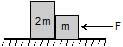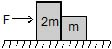Solution:

If F is applied force then acceleration of the system is F/2m+m = F/3m

Now when we apply the force from the left, the force applied on the block m is F/3m = F/3. This will be the force in the contact.

When we apply the force from the right from on the block will be 2Fm/3 = 2F/3, this will be the force on the contact then.

So the ratio is F/3 : 2F/3

= 1 : 3

QUESTION: 22

A body of mass 8 kg is hanging another body of mass 12 kg. The combination is being pulled by a string with an acceleration of 2.2 m s-2. The tension T1 and T2 will be respectively : (use g = 9.8 m/s2)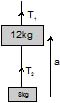Solution:

The tension T1 and T2 will be 240 N, 96 N.
Mass of the body = m1 = 12kg (Given)
Mass of the body = m2 = 8kg (Given)
Acceleration of the string - 2.2m/s² (Given)
Considering positive direction upwards -
Thus,
T1− (m1+m2)g = (m1+m2)a
T1 = (m1+m2)g + (m1+m2)a
T1= (12+8)(2.2)+(12+8)(9.8)
T1 = 44 + 196
= 240 N
T2− (m2)g=(m2)a
T2=(m2)g+(m2)a
T2= (8)(2.2)+(8)(9.8)
T2 = 17.6 + 78.4
= 96 N

QUESTION: 23

A rope of mass 5 kg is moving vertically in vertical position with an upwards force of 100 N acting at the upper end and a downwards force of 70 N acting at the lower end. The tension at midpoint of the rope is

Solution:

When you are given one upward and one downward force on a rope of some mass, and you have to find the tension at the mid- point, then you can simply average the 2 forces. So, the correct answer is (100+70)/3 = 85N

QUESTION: 24

A particle of small mass m is joined to a very heavy body by a light string passing over a light pulley. Both bodies are free to move. The total downward force in the pulley is

Solution:

The heavy body will fall due to accelaration due to gravity.The accelaration of the lighter body wil be 'g' in upward direction.Taking the equlibrium of the small body

T-mg=ma

but a=g

Therefore

T-mg=mg

T=2mg

reation at the pulley

2T=4mg

QUESTION: 25

The pulley arrangements shown in figure are identical the mass of the rope being negligible. In case I, the mass m is lifted by attaching a mass 2 m to the other end of the rope. In case II, the mass m is lifted by pulling the other end of the rope with cosntant downward force F = 2 mg, where g is acceleration due to gravity. The acceleration of mass in case I is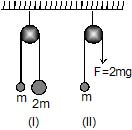Solution:

When we make the free body diagram of two mass system in case 1, we get the equation
2mg - mg = (2m + m) a
Thus we get a = g/3
But in the case 2 the equations is as follows
2mg - mg = ma
Thus we get a - g here.

QUESTION: 26

Two masses M1 and M2 are attached to the ends of a light string which passes over a massless pulley attached to the top of a double inclined smooth plane of angles of inclination a and b. The tension in the string is: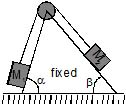Solution:

If we straighten the string horizontally hypothetically and make the free body diagram for the whole two block system we get that
M1g.sinɑ - M2g.sinβ = (M1 + M2)a
For some net acceleration of the system a
Such that a = M1g.sinɑ - M2g.sinβ / (M1 + M2)
Hence if we make a free body diagram of mass 1 only we get
M1g.sinɑ - T = M1a
Thus we get T = M1 ( g.sinɑ - a )
= M1( g.sinɑ - [M1g.sinɑ - M2g.sinβ / (M1 + M2) ] )
= M1( M2 (sinɑ + sinβ )f / (M1 + M2) )

QUESTION: 27

Two masses are hanging vertically over frictionless pulley. The acceleration of the two masses is-

Solution:
QUESTION: 28

Three equal weights A, B, C of mass 2 kg each are hanging on a string passing over a fixed frictionless pulley as shown in the fig. The tension in the string connecting weights B and C is-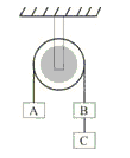Solution:

For some time let us consider B and C to be one single block of mass 4kg. Now if we make the free body diagram for blocks A, B, C we get the net acceleration of the system by equation
4.g - 2.g = 6.a
Thus we get a= g/3
Now if we only make a fbd of block C, we get
2.g - T = 2.a
Thus T = 2 (g - g/3)
= 4g/3
= 13.3 N

QUESTION: 29

In the arrangement shown in figure, pulleys are massless and frictionless and threads are inextensible. The Block of mass m1 will remain at rest, if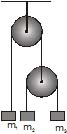Solution: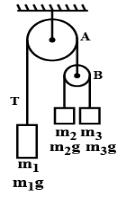a=[(m3​−m2 )/(m2​+m3​) ​​]g      m3>m2
T=[2m2​m3​g]/[m2​+m3] ​
​T′=2T=[4m2​m3​g​]/[m2​+m3​]
m1​g=4m2​m3​g​m/m2​+m3
4/1​=[1/m2​​]+[1/m3​]​​

QUESTION: 30

A block is placed on a rough floor and a horizontal force F is applied on it. The force of friction f by the floor on the block is measured for different values of F and a graph is plotted between them-

Solution: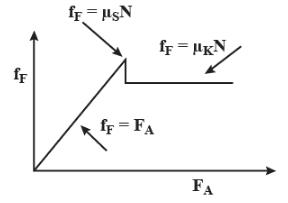For a small value of the applied force the force of friction f increases linearly upto the limiting friction. When it crosses the maximum point of static friction then the friction force due to kinetic friction does not change any more.